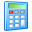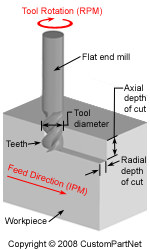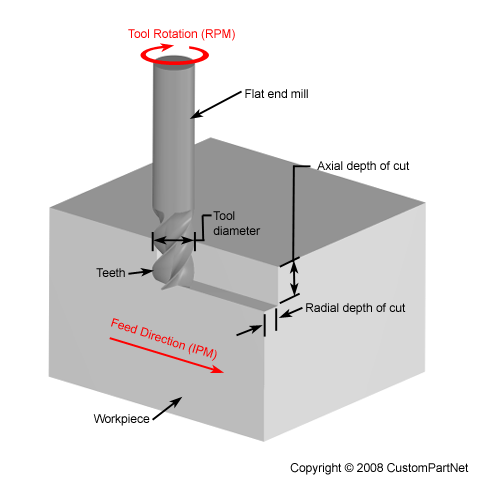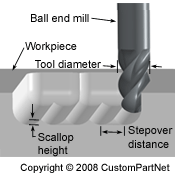Widgets

## Milling Speed and Feed Calculator

 Feed per tooth (IPT) × Number of teeth = Cutting feed (IPR) Cutting speed (SFM) × 12 ÷ Tool diameter (in) × π = Spindle speed (RPM) Cutting feed (IPR) × Spindle speed (RPM) = Feed rate (IPM)
 Cut length (in) ÷ Feed rate (IPM) = Cut time (min)## Milling Horsepower Calculator

 Feed rate (IPM) × Radial depth of cut (in) × Axial depth of cut (in) = Material removal rate (in³/min)
 Material removal rate (in³/min) × Unit power (hp/in³/min) = Spindle horsepower (hp) Spindle horsepower (hp) ÷ Machine efficiency (%) / 100 / 100 = Motor horsepower (hp) Spindle horsepower (hp) × 63,030 × 63,030 ÷ Spindle speed (RPM) = Spindle torque (in∙lb)## Milling Step-over Distance Calculator